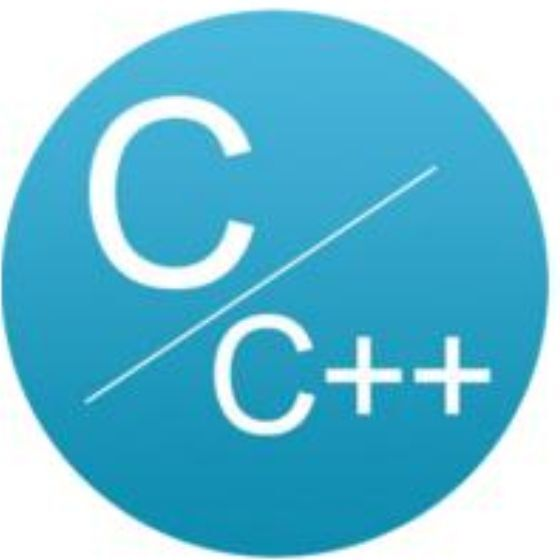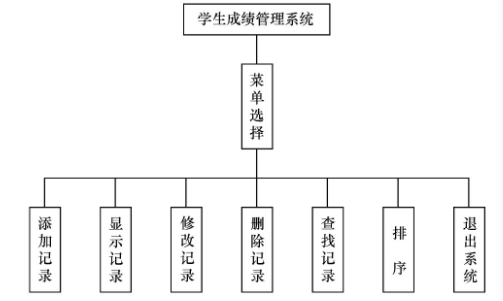# 学生成绩管理系统案例C语言与CPP编程

## 案例描述

1. 添加学生信息，包括学号、姓名、语文成绩、数学成绩；

2. 显示学生信息，将所有学生信息打印输出；

3. 修改学生信息，可以根据姓名查找到学生，然后可以修改学生姓名、成绩项；

4. 删除学生信息，根据学号查找到学生，将其信息删除；

5. 查找学生信息，根据学生姓名，将其信息打印输出；

6. 按学生总成绩进行从高到低排序。## 案例分析

void add(struct student stu[]){    int i, id = 0; //i作为循环变量，id用来保存新学号    char quit;  //保存是否退出的选择    do    {        printf("学号：");        scanf("%d", &id);        for (i = 0; i < n; i++)        {             if (id == stu[i].id)            {                printf("此学号存在！\n");                 return;            }        }               stu[i].id = id;       printf("姓名：");       scanf("%s", &stu[i].name);       printf("计算机基础成绩：");       scanf("%d", &stu[i].chinese);       printf("数据结构成绩：");       scanf("%d", &stu[i].math);       stu[i].sum = stu[i].chinese + stu[i].math;       n++;  //记录条数加1       printf("是否继续添加?(Y/N)");       scanf("\t%c", &quit);    } while (quit != 'N');}

void show(struct student stu[], int i){    printf("%-10d", stu[i].id);    printf("%-10s", stu[i].name);    printf("%-10d", stu[i].chinese);    printf("%-10d", stu[i].math);    printf("%-10d\n", stu[i].sum);}

void modify(struct student stu[]){    char name, ch;    int i;    printf("修改学生的记录。\n");    printf("请输入学生的姓名：");    scanf("%s", &name);    for (i = 0; i < n; i++)    {        if (strcmp(name, stu[i].name) == 0)        {             getchar();             printf("找到该生的记录，如下所示：\n");             HH;  //显示记录的标题             show(stu, i);             printf("是否修改?(Y/N)\n");            scanf("%c", &ch);            if (ch == 'Y' || ch == 'y')            {                 getchar();                 printf("姓名：");                 scanf("%s", &stu[i].name);                 printf("计算机基础成绩：");                 scanf("%d", &stu[i].chinese);                 printf("数据结构成绩：");                 scanf("%d", &stu[i].math);                 stu[i].sum = stu[i].chinese + stu[i].math;                 printf("修改完毕。\n");            }            return;        }    }    printf("没有找到该生的记录。\n");}
1. 删除记录——del()函数当用户在功能菜单中选择数字4时，会调用del()函数进入删除记录模块，对学生学号进行判断，如果学号存在即可删除该生的所有信息，否则输出没有找到该生的记录。

void del(struct student stu[]){    int id, i;    char ch;    printf("删除学生的记录。\n");    printf("请输入学号：");    scanf("%d", &id);    for (i = 0; i < n; i++)    {        if (id == stu[i].id)        {            getchar();            printf("找到该生的记录，如下所示：\n");            HH;  //显示记录的标题            show(stu, i);   //显示数组stu中的第i条记录            printf("是否删除?(Y/N)\n");            scanf("%c", &ch);                        if (ch == 'Y' || ch == 'y')            {                 for (; i < n; i++)                     // 被删除记录后面的记录均前移一位                     stu[i] = stu[i + 1];                     n--;   //记录总条数减1                     printf("删除成功！");            }            return;        }    }    printf("没有找到该生的记录！\n");}

void search(struct student stu[]){    char name;    int i;    printf("查找学生的记录。\n");    printf("请输入学生的姓名：");    scanf("%s", &name);    for (i = 0; i < n; i++)    {        if (strcmp(name, stu[i].name) == 0)        {             printf("找到该生的记录，如下所示：\n");             HH;  //显示记录的标题             show(stu, i); //显示数组stu中的第i条记录            return;        }    }    printf("没有找到该生的记录。\n");}
1. 排序——sort()函数当用户在功能菜单中输入数字6时，会调用sort()函数进入排序记录模块，该模块会输出所有学生的信息，并按总成绩由高到低进行排序。

void sort(struct student stu[]){    int i, j;    struct student t;    printf("按总成绩进行排序，");        for (i = 0; i < n - 1; i++)    {        for (j = i + 1; j < n; j++)        {            if (stu[i].sum < stu[j].sum)            {                 t = stu[i];                 stu[i] = stu[j];                 stu[j] = t;            }        }    }    printf("排序结果如下：\n");    showAll(stu); //显示排序后的所有记录}

## 案例实现

1. 定义student.h文件在student.h文件中定义项目需要的变量与函数声明。在定义变量时，因为学生信息包括学号、姓名和成绩等不同数据类型的属性，所以需要定义一个学生类型的结构体。在存储学生信息时，可选用数组、字符串指针，考虑到学生要根据总成绩来排序，为方便排序，我们选用数组来存储学生信息。

struct student{    int  id;               // 学号    char name;  //姓名    int chinese;     //计算机基础成绩    int math;         //数据结构成绩    int sum;          //总分};
1. 定义student.c文件在student.c文件中实现各个功能函数。

2. 定义main.c文件在main.c文件中，定义保存学生信息的结构体数组，构建学生成绩管理系统主界面，使用while（1）循环控制是否退出系统，在while循环中使用switch语句判断用户所选择的功能，根据用户选择的功能调用相应的函数。

﻿﻿## 评论